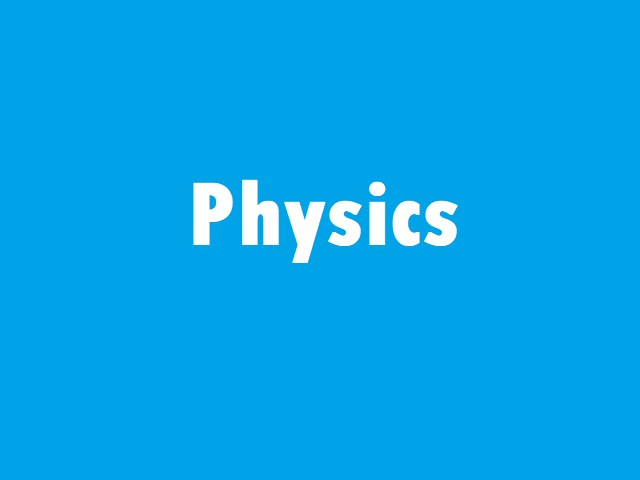Thursday, May 19, 2022
HomeLATEST JOBCBSE 12th Physics Board Exam 2022

# CBSE 12th Physics Board Exam 2022Check Term 2 CBSE Class 12 Physics Syllabus 2022. It is essential for the preparation of the upcoming Term 2 CBSE 12th Physics board exam 2022. Students preparing for Term 2 CBSE Class 12 Physics board exam 2022 should plan their studies accordingly.

## CBSE Class 12 Physics Syllabus 2022 (Term 2): CBSE 12th Physics Board Exam 2022

Class XII Syllabus assigned for Term II (Theory)

Time: 2 Hours Max Marks: 35

 Marks Unit – V Electromagnetic Waves 17 Chapter–8: Electromagnetic Waves Unit – VI Optics Chapter–9: Ray Optics and Optical Instruments Chapter–10: Wave Optics Unit – VII Dual Nature of Radiation and Matter 11 Chapter–11: Dual Nature of Radiation and Matter Unit–VIII Atoms and Nuclei Chapter–12: Atoms Chapter–13: Nuclei Unit IX Electronic Devices 7 Chapter–14: Semiconductor -Electronics: Materials, Devices and Simple Circuits Total 35

Unit V: Electromagnetic waves (2 Periods)

Chapter–8: Electromagnetic Waves

Electromagnetic waves, their characteristics, their Transverse nature (qualitative ideas only).

Electromagnetic spectrum (radio waves, microwaves, infrared, visible, ultraviolet, X-rays, gamma rays) including elementary facts about their uses.

Unit VI: Optics (18 Periods)

Chapter–9: Ray Optics and Optical Instruments

Ray Optics: Refraction of light, total internal reflection and its applications, optical fibers, refraction at spherical surfaces, lenses, thin lens formula, lensmaker’s formula, magnification, power of a lens, combination of thin lenses in contact, refraction of light through a prism.

Optical instruments: Microscopes and astronomical telescopes (reflecting and refracting) and their magnifying powers.

Chapter–10: Wave Optics

Wave optics: Wavefront and Huygens principle, reflection and refraction of plane wave at a plane surface using wave fronts. Proof of laws of reflection and refraction using Huygens principle.

Interference, Young’s double slit experiment and expression for fringe width, coherent sources and sustained interference of light, diffraction due to a single slit, width of central maximum

Unit VII: Dual Nature of Radiation and Matter (7 Periods)

Chapter–11: Dual Nature of Radiation and Matter

Dual nature of radiation, Photoelectric effect, Hertz and Lenard’s observations; Einstein’s

photoelectric equation-particle nature of light.

Experimental study of photoelectric effect Matter waves-wave nature of particles, de-Broglie relation

Unit VIII: Atoms and Nuclei (11 Periods)

Chapter–12: Atoms

Alpha-particle scattering experiment; Rutherford’s model of atom; Bohr model, energy levels, hydrogen spectrum.

Chapter–13:

Nuclei Composition and size of nucleus Nuclear force Mass-energy relation, mass defect, nuclear fission, nuclear fusion.

Unit IX: Electronic Devices (7 Periods)

Chapter–14:

Semiconductor Electronics: Materials, Devices and Simple Circuits Energy bands in conductors, semiconductors and insulators (qualitative ideas only)

Semiconductor diode – I-V characteristics in forward and reverse bias, diode as a rectifier; Special purpose p-n junction diodes:

LED, photodiode, solar cell.

Syllabus assigned for CBSE Class Physics Practical for Term II (Total Periods: 16)

The second term practical examination will be organised by schools as per the directions of CBSE and viva will be taken by both internal and external observers. The record to be submitted by the students at the time of second term examination has to include a record of at least 4 Experiments and 3 Activities to be demonstrated by the teacher.

Evaluation Scheme

Time Allowed: one and half hours: Max. Marks: 15

Two experiments to be performed by students at time of examination: 8 marks

Practical record [experiments and activities]: 2 marks

Viva on experiments, and activities: 5 marks

Total: 15 marks

Experiments assigned for Term-II

1. To find the focal length of a convex lens by plotting graphs between u and v or between 1/u and 1/v.

2. To find the focal length of a convex mirror, using a convex lens.

OR

To find the focal length of a concave lens, using a convex lens.

3. To determine angle of minimum deviation for a given prism by plotting a graph between angle of incidence and angle of deviation.

4. To determine the refractive index of a glass slab using a travelling microscope.

5. To find the refractive index of a liquid by using a convex lens and plane mirror.

6. To draw the I-V characteristic curve for a p-n junction diode in forward bias and reverse bias.

Activities assigned for Term-II

1. To identify a diode, an LED, a resistor and a capacitor from a mixed collection of such items.

2. Use of multimeter to see the unidirectional flow of current in case of a diode and an LED and

check whether a given electronic component (e.g., diode) is in working order.

3. To study effect of intensity of light (by varying distance of the source) on an LDR.

4. To observe refraction and lateral deviation of a beam of light incident obliquely on a glass slab.

5. To observe polarization of light using two Polaroids.

6. To observe diffraction of light due to a thin slit.

7. To study the nature and size of the image formed by a (i) convex lens, (ii) concave mirror, on a screen by using a candle and a screen (for different distances of the candle from the

lens/mirror).

8. To obtain a lens combination with the specified focal length by using two lenses from the given set of lenses.

RELATED ARTICLES

Fans
Followers
0Followers
Followers
Subscribers